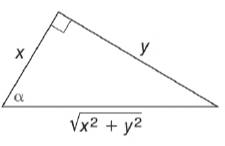Chapter 11.3, Problem 8EElementary Geometry For College St...

7th Edition
Alexander + 2 others
ISBN: 9781337614085

Solutions

Chapter
SectionElementary Geometry For College St...

7th Edition
Alexander + 2 others
ISBN: 9781337614085
Textbook Problem

In Exercises 5 to 10, find the value (or expression) for each of the six trigonometric ratios of angle α . Use the Pythagorean Theorem as needed.To determine

To find:

The value for each of the six trigonometric ratios of angle α.

Explanation

Given:

The given figure is

Definition used:

The six trigonometric ratios:

Calculation:

From the figure, opposite=y, adjacent=x, hypotenuse=x2+y2.

Still sussing out bartleby?

Check out a sample textbook solution.

See a sample solution

The Solution to Your Study Problems

Bartleby provides explanations to thousands of textbook problems written by our experts, many with advanced degrees!

Get Started

Subtract and check: 417,286287,156

Elementary Technical Mathematics

Evaluate 111+x4dx

Calculus (MindTap Course List)

23/2

Applied Calculus for the Managerial, Life, and Social Sciences: A Brief Approach

Define the alpha level and the critical region for a hypothesis test.

Statistics for The Behavioral Sciences (MindTap Course List)

Multiply. (a2)(a3) (sin2)(sin3)

Trigonometry (MindTap Course List)

True or False: The function whose graph is given at the right is a probability density function.

Study Guide for Stewart's Single Variable Calculus: Early Transcendentals, 8th

The length of the are r = eθ for 0 ≤ θ ≤ π is given by:

Study Guide for Stewart's Multivariable Calculus, 8th